# Pythagoras Theorem

Pythagoras Theorem
Go back to  'Triangles'

 1 What is Pythagoras Theorem? 2 Pythagoras Theorem Statement 3 Pythagoras Theorem Derivation 4 Pythagoras Theorem Formula 5 Pythagorean Theorem Calculator 6 Important Notes on Pythagoras Theorem 7 Solved Examples on Pythagoras Theorem 8 Practice Questions on Pythagoras Theorem 9 Common Misconceptions 10 Challenging Questions 11 Maths Olympiad Sample Papers 12 Frequently Asked Questions (FAQs) 13 FREE Worksheets of Pythagoras Theorem

We at Cuemath believe that Math is a life skill. Our Math Experts focus on the “Why” behind the “What.” Students can explore from a huge range of interactive worksheets, visuals, simulations, practice tests, and more to understand a concept in depth.

Book a FREE trial class today! and experience Cuemath's LIVE Online Class with your child.

## What is Pythagoras Theorem?

Pythagoras was a Greek philosopher born in 571 BCE in Samos, Greece.

The Pythagoras theorem definition shows the relation among the three sides of a right triangle.

The square of the hypotenuse is equal to the sum of the square of the other two sides.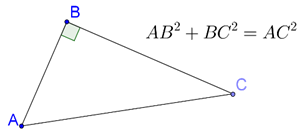Right triangles follow this rule and they are called Pythagoras theorem triangle.

## Pythagoras Theorem Statement

In a right-angled triangle, the square of the hypotenuse is equal to the sum of the squares of the other two sides.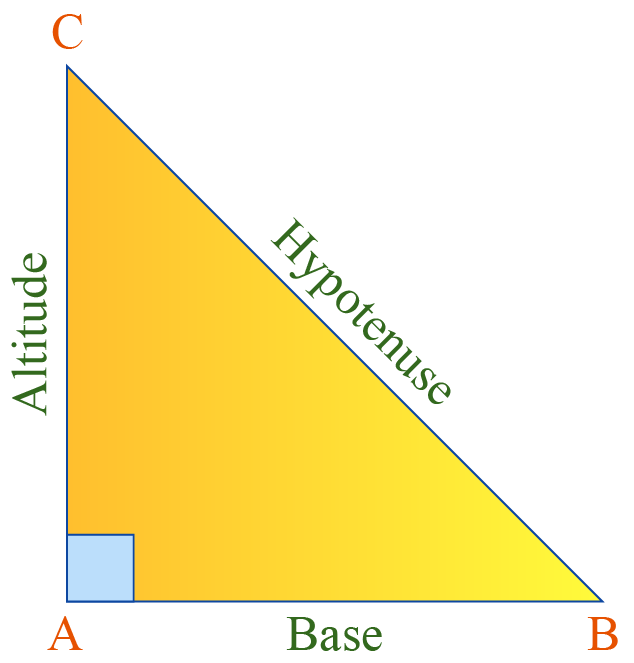In the given $$\Delta ABC$$, we see

\begin{align} \text{BC}^2 &= \text{AB}^2 +\text{AC}^2 \end{align}

where,

• $$\text{AB}$$ is the base,
• $$\text{AC}$$ is the altitude or the height, and
• $$\text{BC}$$ is the hypotenuse.

Hence, the Pythagoras Theorem states that:

 \begin{align} \text{Hypotenuse}^2 &= \text{Base}^2 +\text{Altitude}^2 \end{align}

Let's explore this with the help of a simulation.

Click Go to see the Geometric proof of the Pythagoras Theorem:

CLUEless in Math? Check out how CUEMATH Teachers will explain Pythagoras Theorem to your kid using interactive simulations & worksheets so they never have to memorise anything in Math again!

Explore Cuemath Live, Interactive & Personalised Online Classes to make your kid a Math Expert. Book a FREE trial class today!

## Pythagoras Theorem Derivation

The Pythagoras theorem definition can be derived and proved in different ways. Let us see a few methods here.

### By Algebraic method

Consider four right triangles $$\Delta ABC$$ where b is the base, a is the height and c is the hypotenuse.

Arrange these four congruent right triangles in the given square, whose side is ($$\text {a + b}$$).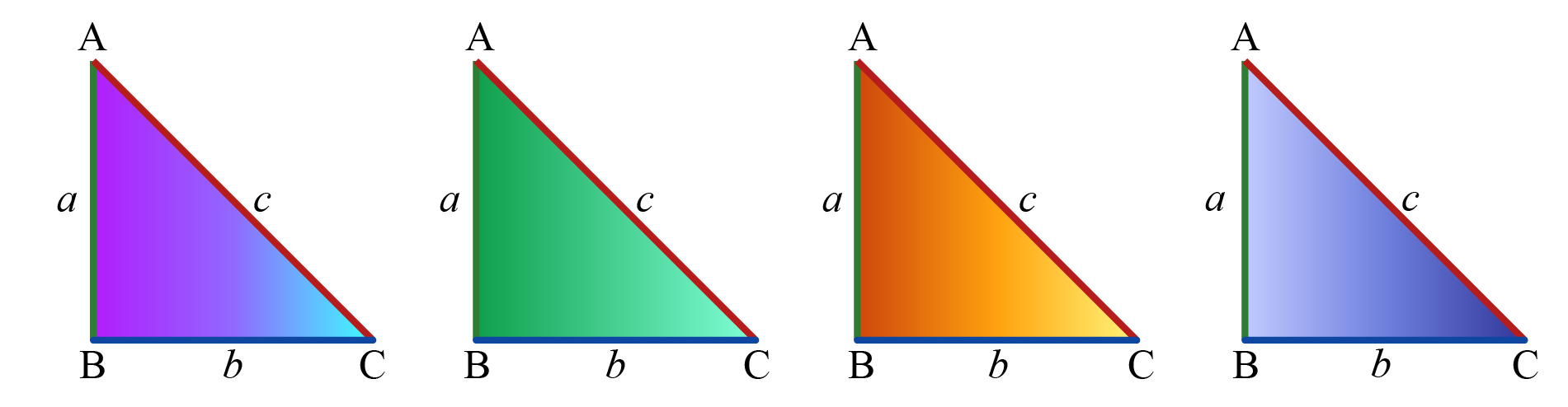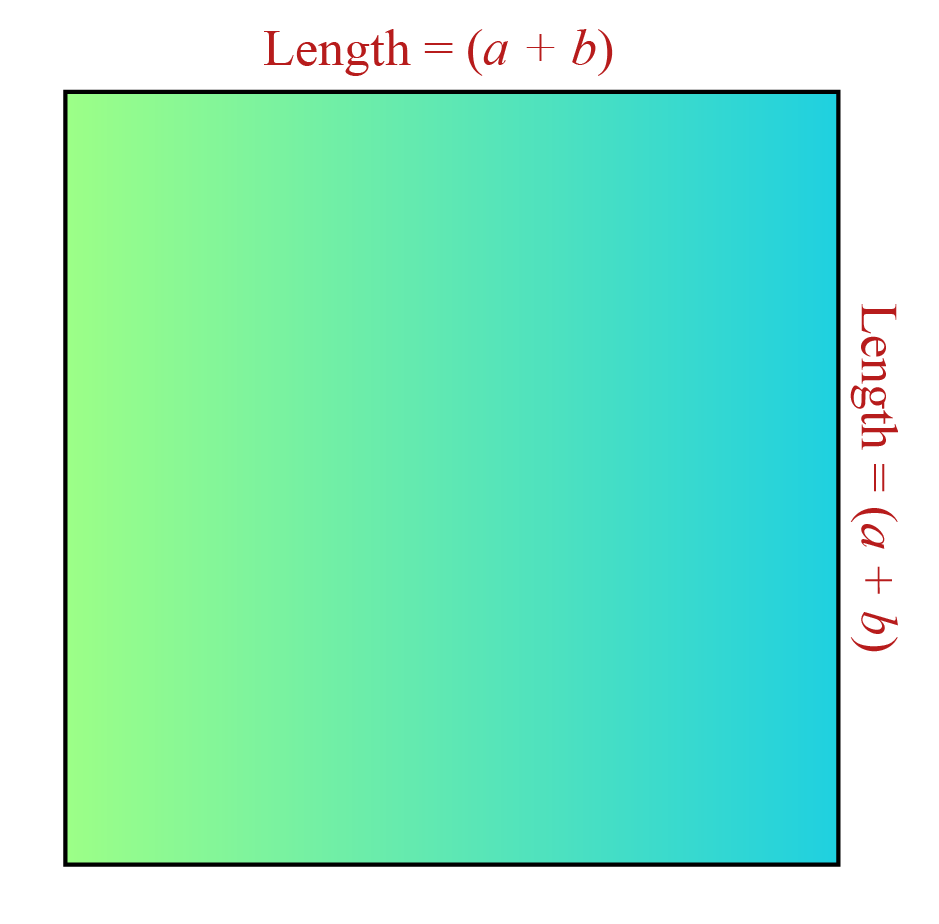Start the simulation below to observe how these congruent triangles are placed and how the proof of the Pythagoras theorem is derived using the algebraic method.

### By Similarity of Triangles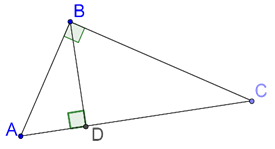$$\Delta ABD$$ and $$\Delta ACB$$:

• $$\angle A = \angle A$$ (common)
• $$\angle ADB = \angle ABC$$ (both are right angles)

Thus, $$\Delta ABD$$ and $$\Delta ACB$$ are equi-angular, which means that they are similar by AA similarity criterion.

Similarly, we can prove

$\Delta BCD\sim \Delta ACB$

(Note carefully the order of the vertices)

Since

$\Delta ABD \sim \Delta ACB$

we have:

\begin{align} \frac{{AD}}{{AB}} &= \frac{{AB}}{{AC}} \hfill \\\\ \Rightarrow AD \times AC &= A{B^2}....(1) \hfill \\ \end{align}

Similarly,

$\Delta BCD \sim \Delta ACB$

gives:

\begin{align}\frac{{CD}}{{BC}} &= \frac{{BC}}{{AC}} \\\\ \Rightarrow CD \times AC &= B{C^2}....(2)\end{align}

Adding (1) and (2), we have:

$\begin{array}{*{20}{l}} {\left( {AD \times AC} \right) + \left( {CD \times AC} \right) = A{B^2} + B{C^2}} \\\\ { \Rightarrow (AD + CD) \times AC = A{B^2} + B{C^2}} \\\\ { \Rightarrow \boxed{A{C^2} = A{B^2} + B{C^2}}\left( {\because\! AD \!+\! CD \!=\! AC} \right)} \end{array}$

## Formula - Pythagoras Theorem

The Pythagoras theorem formula states that in a right triangle $$\text {ABC}$$, the square of the hypotenuse is equal to the sum of the square of the other two legs.$\text{AB}^2 + \text{AC}^2 =\text{BC}^2$

where,

• $$\text{AB}$$ is the base
• $$\text{AC}$$ is the altitude or the height  and
• $$\text{BC}$$ is the hypotenuse.

You can refer to the Solved Examples section here for some interesting real-life pythagoras' theorem questions.

## Pythagorean Theorem Calculator

Use this Pythagoras theorem calculator to see the relationship between the altitude, height and hypotenuse of the right triangle.

Key in any two values and see the third value being populated in the right triangle with this measure.

Similar Triangles
grade 9 | Questions Set 2
Similar Triangles
Similar Triangles
grade 9 | Questions Set 1
grade 9 | Questions Set 1
grade 9 | Questions Set 2